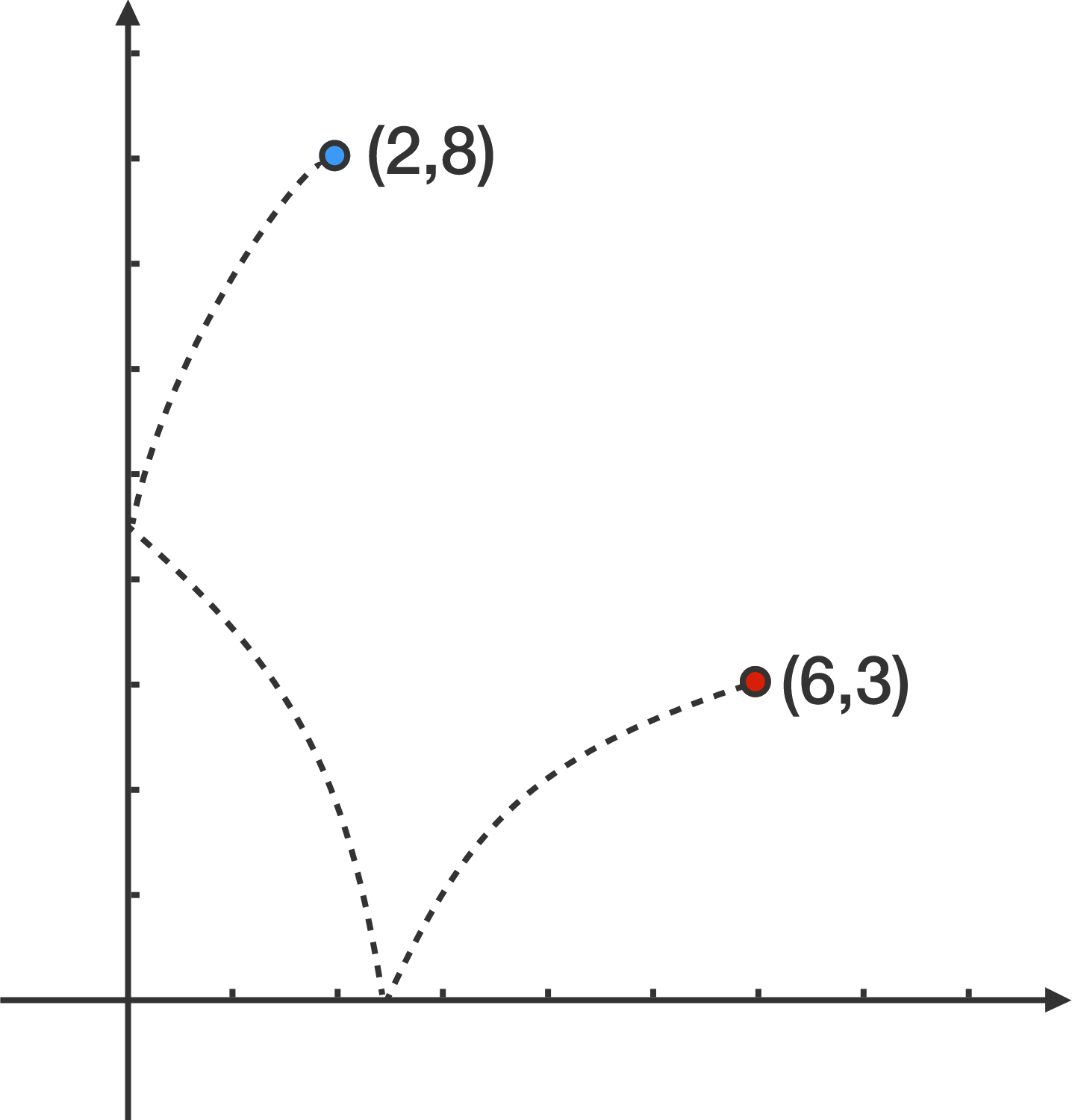# Double Tag

Geometry Level 2Find the length of the shortest path that can be drawn from the point $(6, 3)$ to the point $(2, 8)$ such that the path touches the $x$-axis and the $y$-axis once.

×